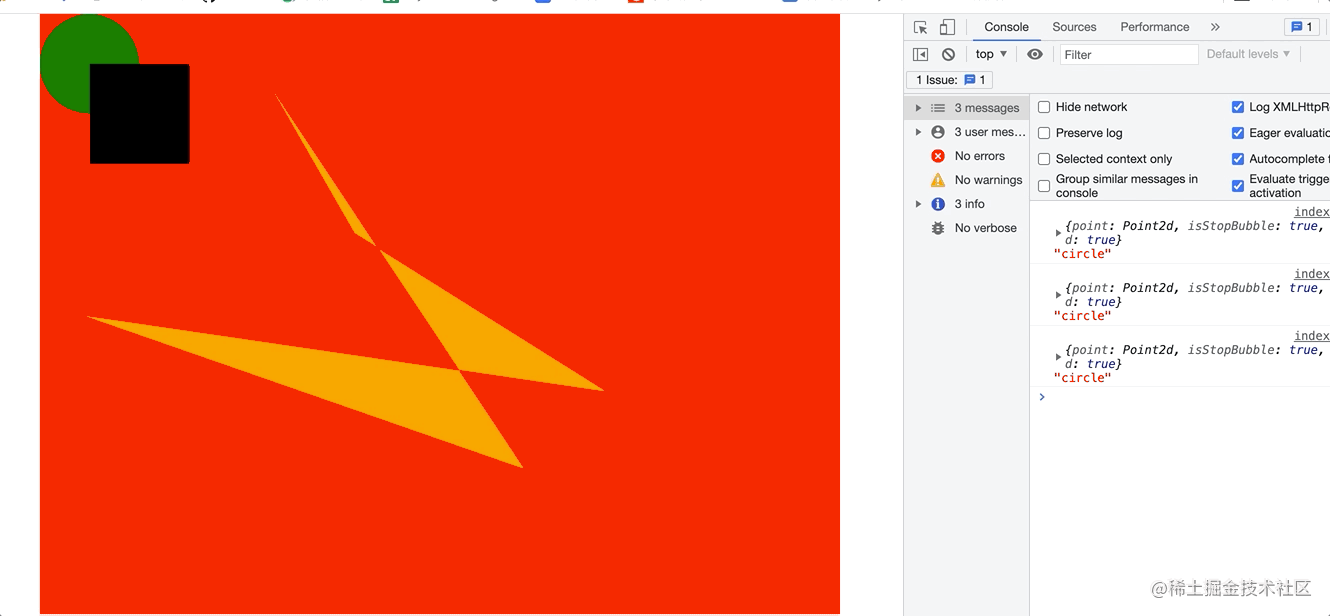# canvas也能实现事件系统？？？？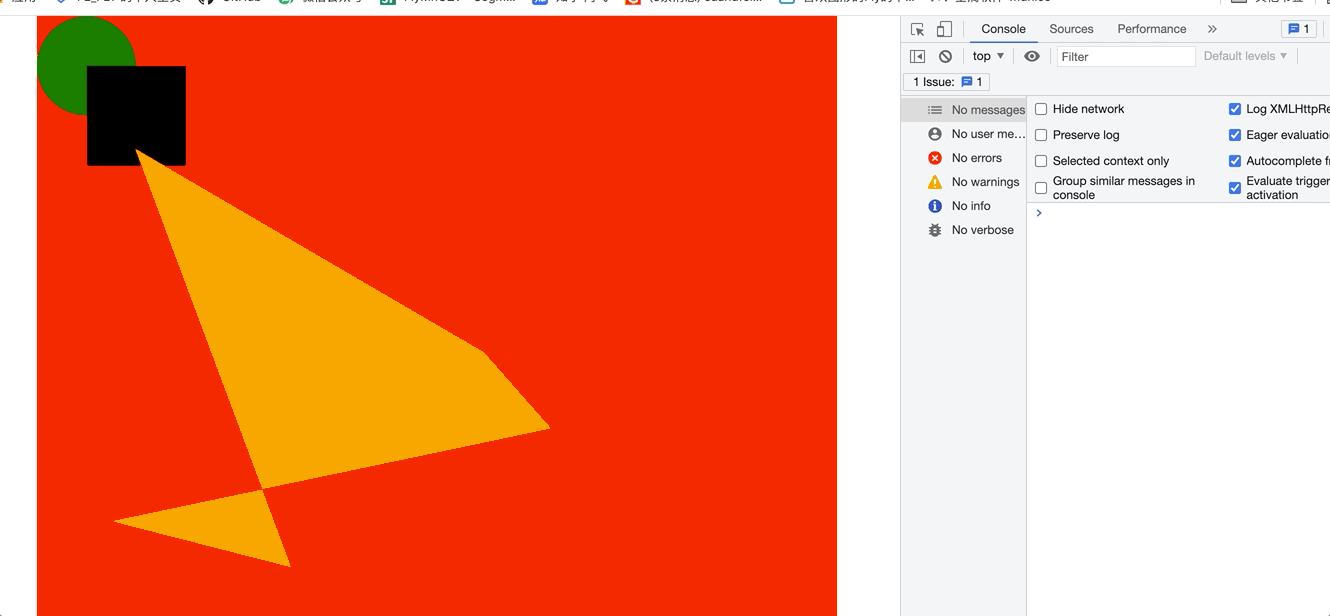# 前言

1. 我是怎么基于canvas去构建基础框架
2. 几何算法—— 判断点是不是任意多边形内部
3. 如何进行事件分发阻止事件冒泡

# 基础框架的搭建

## 图形类

draw 方法， 还有一个方法就是判断鼠标的点 是不是在当前图形的内部，这个我我们后面在讨论吧。 然后每个图形有自己的特有的属性，结合canvas 的api 去设置。

``````export class Circle extends Shape {
constructor(props) {
super()
this.props = props
}
​
draw(ctx) {
}
​
// 判断鼠标的点是否在图形内部
isPointInClosedRegion(mouse) {
}
}
​
export class Rect extends Shape {
constructor(props) {
super()
this.props = props
}
draw(ctx) {
}
​
// 判断鼠标的点是否在图形内部
isPointInClosedRegion(mouse) {
}
}

``````const { center, radius, fillColor = 'black' } = this.props
const { x, y } = center
ctx.save()
ctx.beginPath()
ctx.fillStyle = fillColor
ctx.arc(x, y, radius, 0, Math.PI * 2)
ctx.fill()
ctx.closePath()
ctx.restore()

``````const { leftTop, width, height, fillColor = 'black' } = this.props
const { x, y } = leftTop
ctx.save()
ctx.beginPath()
ctx.fillStyle = fillColor
ctx.fillRect(x, y, width, height)
ctx.closePath()
ctx.restore()

## 画布类

``````// 新建一个画布类
export class Canvas {
constructor() {
this.canvas = document.getElementById('canvas')
this.ctx = this.canvas.getContext('2d')
this.allShapes = []
}
​
shape.draw(this.ctx)
this.allShapes.push(shape)
}
}

``````const canvas = new Canvas()
const circle = new Circle({
center: new Point2d(50, 50),
fillColor: 'green',
})
const rect = new Rect({
leftTop: new Point2d(50, 50),
width: 100,
height: 100,
fillColor: 'black',
})
// 添加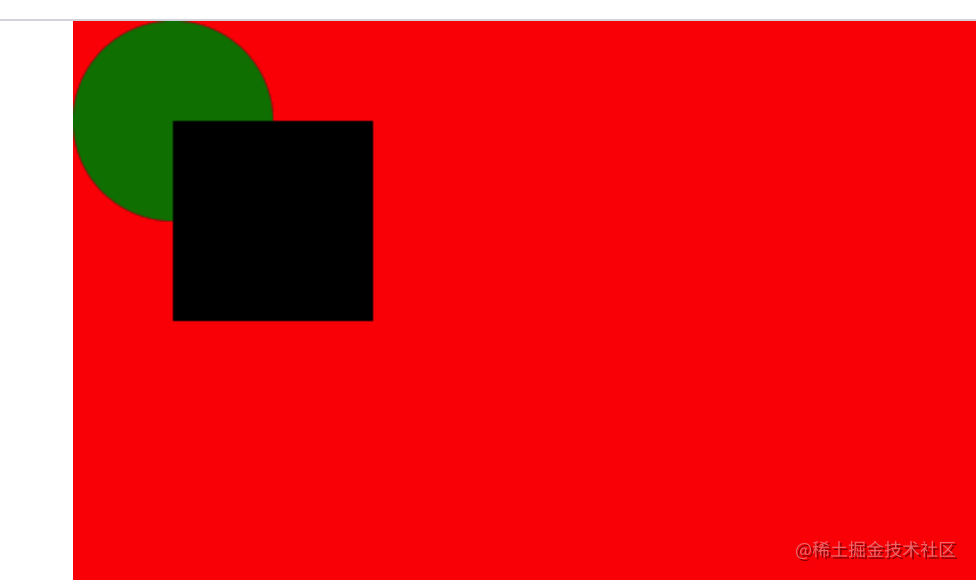OK，看来我们写的代码是没有问题的，下面写一个稍微复杂的图形，任意点组成的闭合polygon

## POLYGON类

``````export class Polygon extends Shape {
constructor(props) {
super()
this.props = props
}
draw(ctx) {
const { points, fillColor = 'black' } = this.props
ctx.save()
ctx.beginPath()
ctx.fillStyle = fillColor
points.forEach((point, index) => {
const { x, y } = point
if (index === 0) {
ctx.moveTo(x, y)
} else {
ctx.lineTo(x, y)
}
})
ctx.fill()
ctx.closePath()
ctx.restore()
}
​
getDispersed() {
return this.props.points
}
​
isPointInClosedRegion(event) {
}
}

``````const points = []
for (let i = 0; i < 5; i++) {
points.push(Point2d.random(800, 600))
}
const shape = new Polygon({
points,
fillColor: 'orange',
})
// 添加到画布中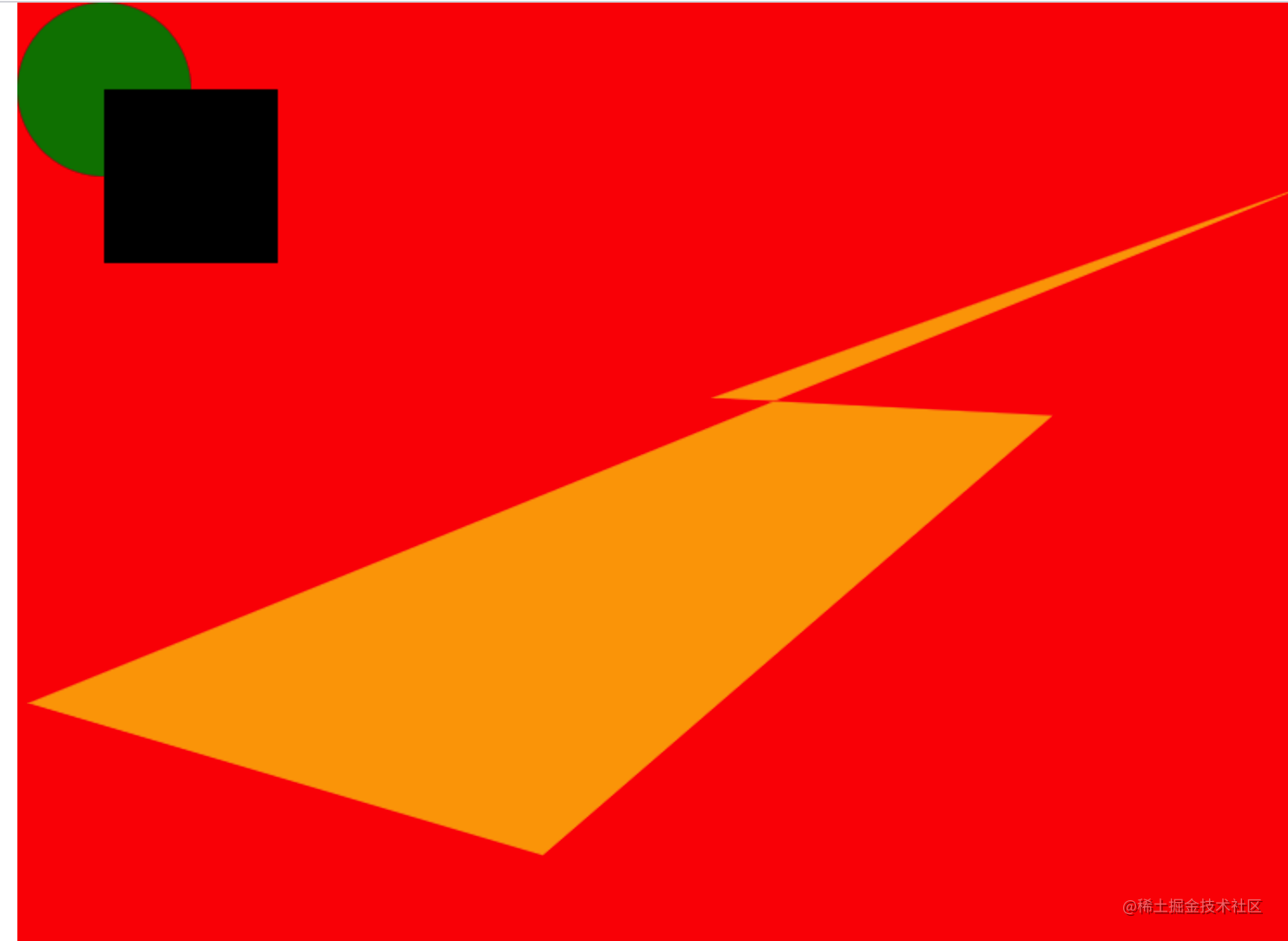## 基类SHAPE

``````// 图形的基类
export class Shape {
constructor() {
this.listenerMap = new Map()
}
on(eventName, listener) {
if (this.listenerMap.has(eventName)) {
this.listenerMap.get(eventName).push(listener)
} else {
this.listenerMap.set(eventName, [listener])
}
}
}

On 这个方法哈， 第一个参数是事件名字， 第二个参数就是listener了， OK到目前为止， 每个图形对应的事件，都有了listener。

# 事件分发

``````this.canvas.addEventListener(move, this.handleEvent(move))

Move 和click 是我定义个两个常量哈：

``````export const move = 'mousemove'
export const click = 'mousedown'

handleEvent 这个方法 用到了函数式编程， 将事件名字 和逻辑 进行解耦哇。

``````handleEvent = (name) => (event) => {
this.allShapes.forEach((shape) => {
// 获取当前事件的所有监听者
const listerns = shape.listenerMap.get(name)
if ( listerns ) {
listerns.forEach((listener) => listener(event))
}
})
}

``````circle.on(click, (event) => {
//event.isStopBubble = true
console.log(event, 'circle')
})
rect.on(click, (event) => {
console.log(event, 'rect')
})## 圆的实现

``````const { center, radius } = this.props

## 矩形的实现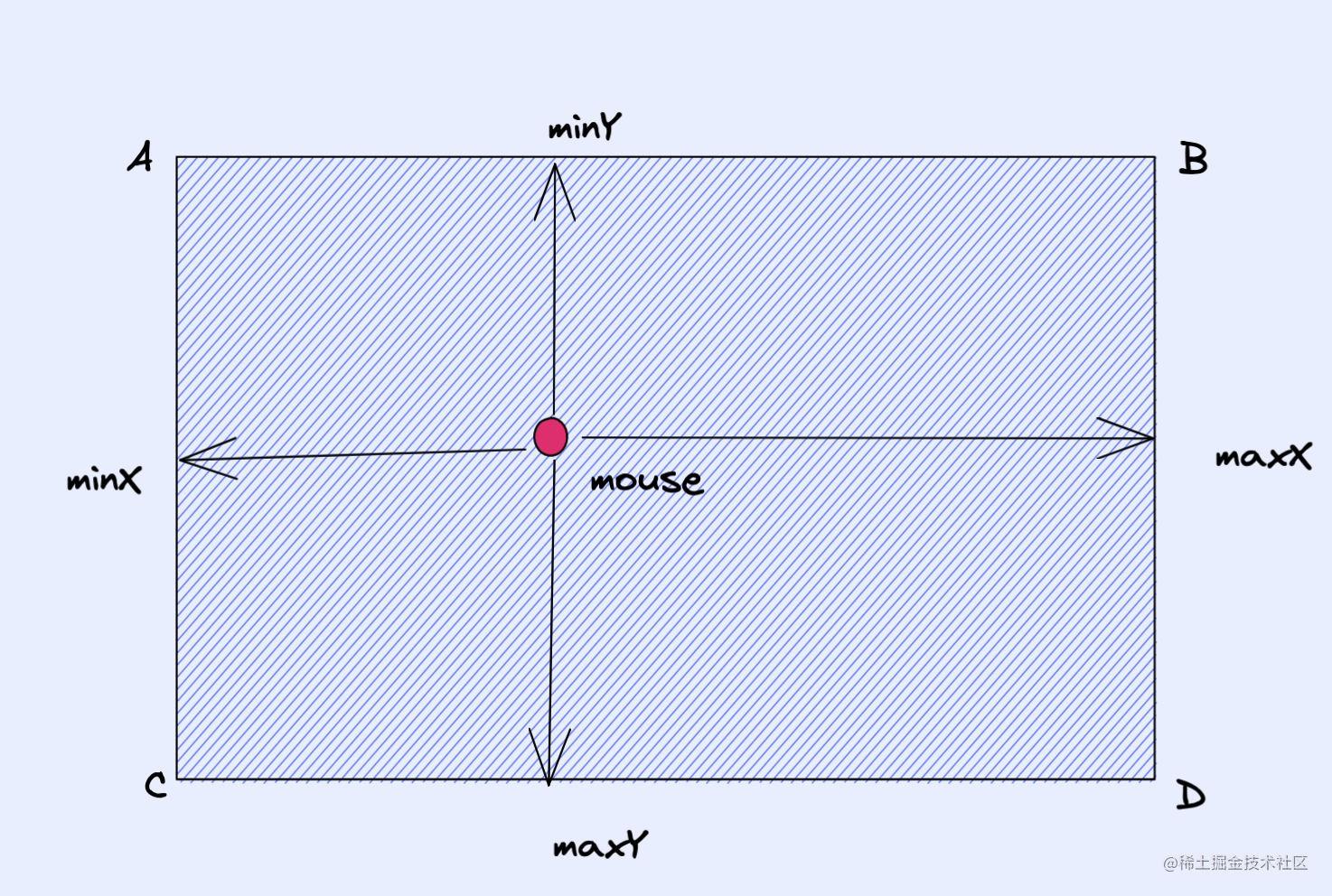``````  // 判断鼠标的点是否在图形内部
isPointInClosedRegion(mouse) {
const { x, y } = mouse.point
const { leftTop, width, height } = this.props
const { x: minX, y: minY } = leftTop
const maxX = minX + width
const maxY = minY + height
if (x >= minX && x <= maxX && y >= minY && y <= maxY) {
return true
}
return false
}

# 点在任意多边形内（算法）

1. 引射线法：从目标点出发引一条射线，看这条射线和多边形所有边的交点数目。如果有奇数个交点，则说明在内部，如果有偶数个交点，则说明在外部。
2. 面积和判别法：判断目标点与多边形的每条边组成的三角形面积和是否等于该多边形，相等则在多边形内部。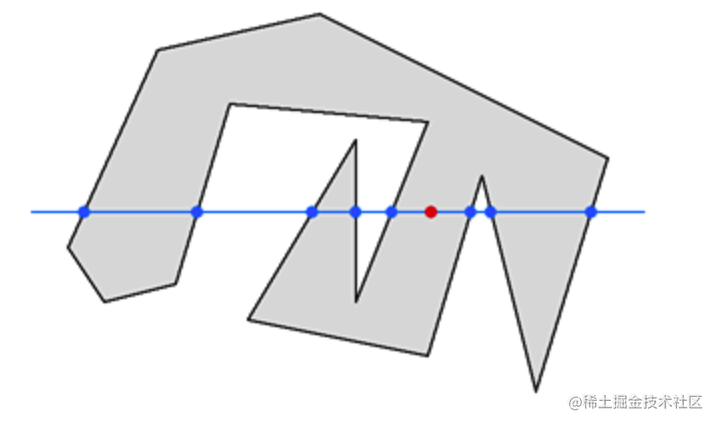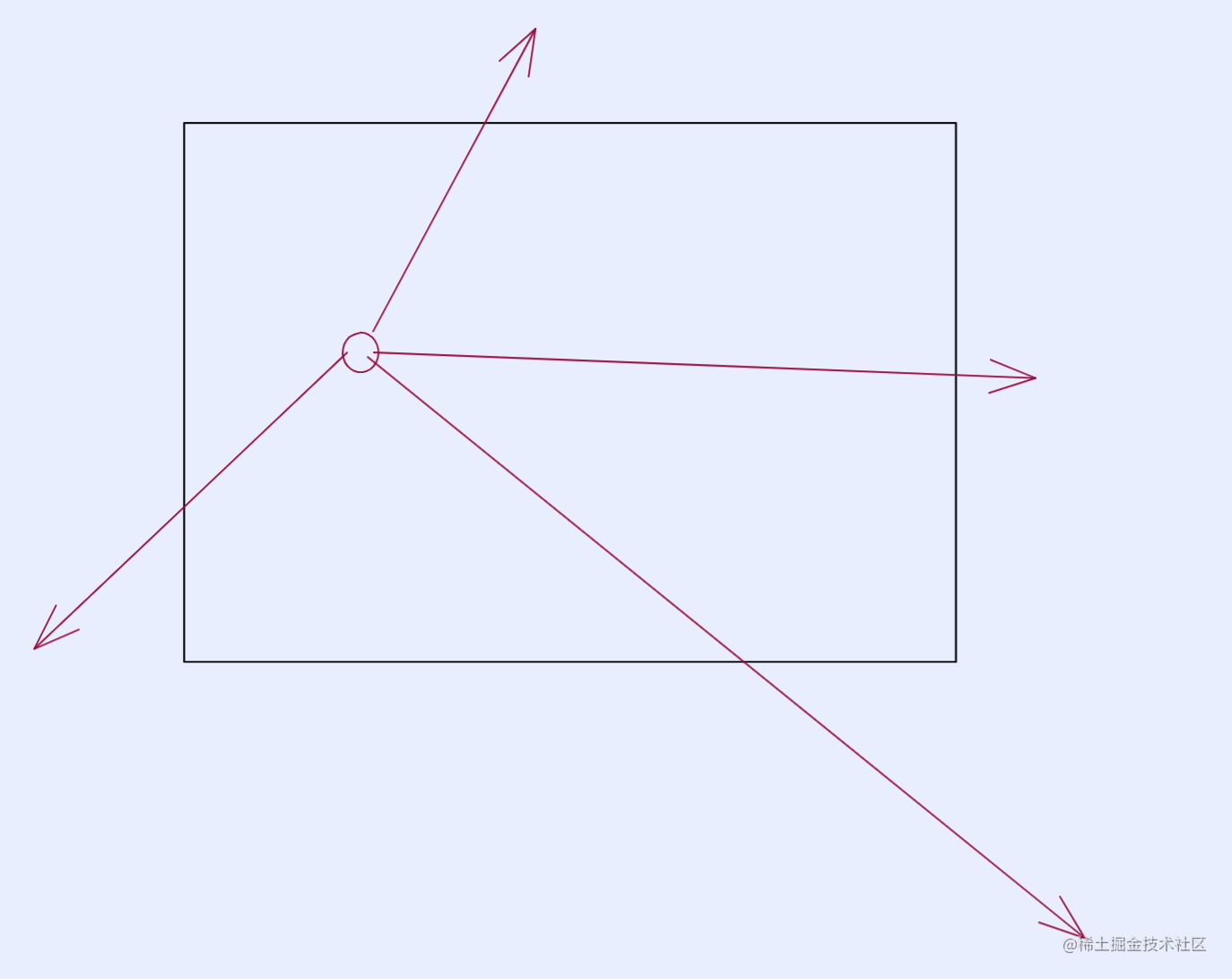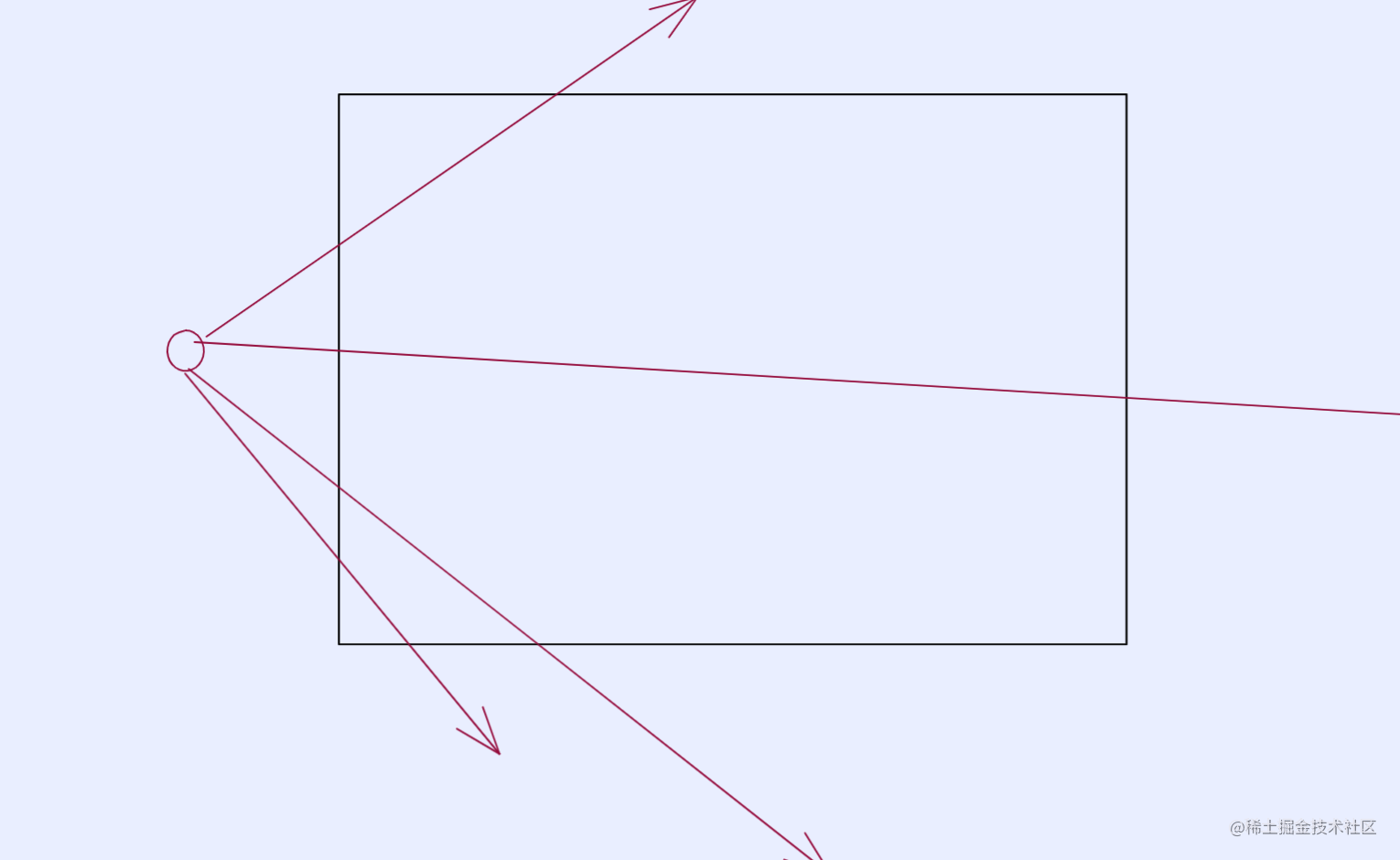## 算法实现

``````export class Seg2d {
constructor(start, end) {
this.endPoints = [start, end]
this._asVector = undefined
}
​
get start() {
return this.endPoints
}
get end() {
return this.endPoints
}
reverse() {
return new Seg2d(this.end.clone(), this.start.clone())
}
clone() {
return new Seg2d(this.start.clone(), this.end.clone())
}
​
get asVector() {
return (
this._asVector ||
(this._asVector = new Point2d(
this.endPoints.x - this.endPoints.x,
this.endPoints.y - this.endPoints.y
))
)
}
​
}

1. 多个点转成线段
2. 线段和线段相交

`````` //一堆点 获得闭合一堆线段
static getSegments(points, closed = false) {
const list = []
for (let i = 1; i < points.length; i++) {
list.push(new Seg2d(points[i - 1], points[i]))
}
if (closed && !points.equal(points[points.length - 1])) {
list.push(new Seg2d(points[points.length - 1], points))
}
return list
}

Closed 这个参数， 因为区域是满足一个方向的。所以闭合区域 肯定是首尾相连的。

## 线段和线段求焦点

1. 列方程求两个直线的焦点
2. 判断每一条线段的两个端点是否都在另一条线段的两侧, 是则求出两条线段所在直线的交点, 否则不相交.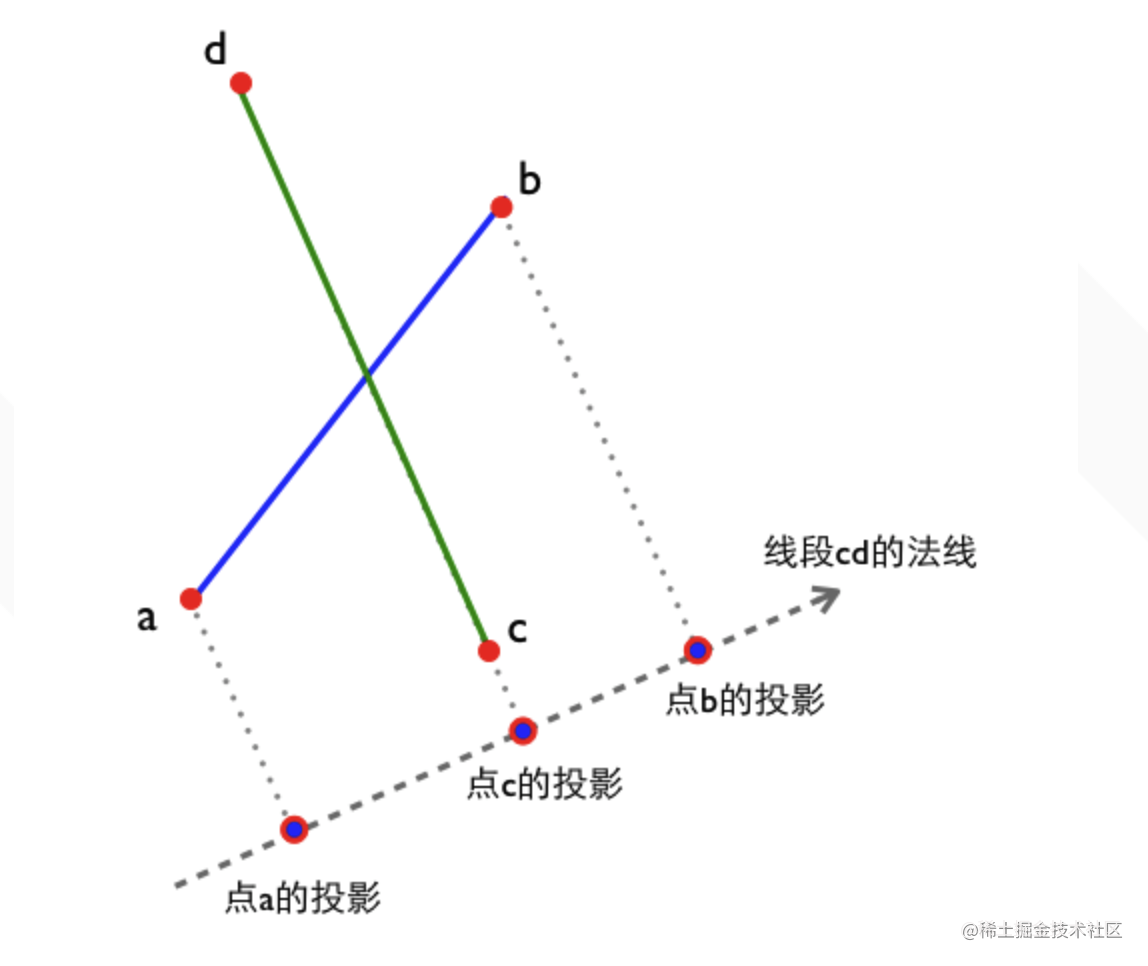``````const nx=b.y - a.y,
ny=a.x - b.x;
const normalLine = {  x: nx, y: ny };

``````const dist= normalLine.x*c.x + normalLine.y*c.y;

distA==distB==distC 时, 两条线段共线 distA==distB!=distC 时, 两条线段平行 distA 和 distB 在distC 同侧时, 两条线段不相交. distA 和 distB 在distC 异侧时, 两条线段是否相交需要再判断点c点d与线段ab的关系.

``````static lineLineIntersect(line1, line2) {
const a = line1.start
const b = line1.end
const c = line2.start
const d = line2.end
const interInfo = []
//线段ab的法线N1
const nx1 = b.y - a.y,
ny1 = a.x - b.x
​
//线段cd的法线N2
const nx2 = d.y - c.y,
ny2 = c.x - d.x
​
//两条法线做叉乘, 如果结果为0, 说明线段ab和线段cd平行或共线,不相交
const denominator = nx1 * ny2 - ny1 * nx2
if (denominator == 0) {
return interInfo
}
​
//在法线N2上的投影
const distC_N2 = nx2 * c.x + ny2 * c.y
const distA_N2 = nx2 * a.x + ny2 * a.y - distC_N2
const distB_N2 = nx2 * b.x + ny2 * b.y - distC_N2
​
// 点a投影和点b投影在点c投影同侧 (对点在线段上的情况,本例当作不相交处理);
if (distA_N2 * distB_N2 >= 0) {
return interInfo
}
​
//
//判断点c点d 和线段ab的关系, 原理同上
//
//在法线N1上的投影
const distA_N1 = nx1 * a.x + ny1 * a.y
const distC_N1 = nx1 * c.x + ny1 * c.y - distA_N1
const distD_N1 = nx1 * d.x + ny1 * d.y - distA_N1
if (distC_N1 * distD_N1 >= 0) {
return interInfo
}
​
//计算交点坐标
const fraction = distA_N2 / denominator
const dx = fraction * ny1,
dy = -fraction * nx1
​
interInfo.push(new Point2d(a.x + dx, a.y + dy))
return interInfo
}

``````isPointInClosedRegion(event) {
const allSegs = Seg2d.getSegments(this.getDispersed(), true)
// 选取任意一条射线
const start = event.point
const xAxias = new Point2d(1, 0).multiplyScalar(800)
const anyRaySeg = new Seg2d(start, end)
let total = 0
allSegs.forEach((item) => {
const intersetSegs = Seg2d.lineLineIntersect(item, anyRaySeg)
total += intersetSegs.length
})
// 奇数在内部
if (total % 2 === 1) {
return true
}
return false
}

OK， 这时候我们吧触发事件的条件改写下。

``````handleEvent = (name) => (event) => {
this.allShapes.forEach((shape) => {
// 获取当前事件的所有监听者
const listerns = shape.listenerMap.get(name)
if (
listerns &&
shape.isPointInClosedRegion(event)
) {
listerns.forEach((listener) => listener(event))
}
})
}

# 阻止事件冒泡

1. 第一件事就是将鼠标的点 转为 point2d
2. 增加一个属性isStopBubble 来阻止冒泡

`````` getNewEvent(event) {
const point = new Point2d(event.offsetX, event.offsetY)
return {
point,
isStopBubble: false,
...event,
}
}

``````handleEvent = (name) => (event) => {
event = this.getNewEvent(event)
this.allShapes.forEach((shape) => {
// 获取当前事件的所有监听者
const listerns = shape.listenerMap.get(name)
if (
listerns &&
shape.isPointInClosedRegion(event)
&& !event.isStopBubble
) {
listerns.forEach((listener) => listener(event))
}
})
}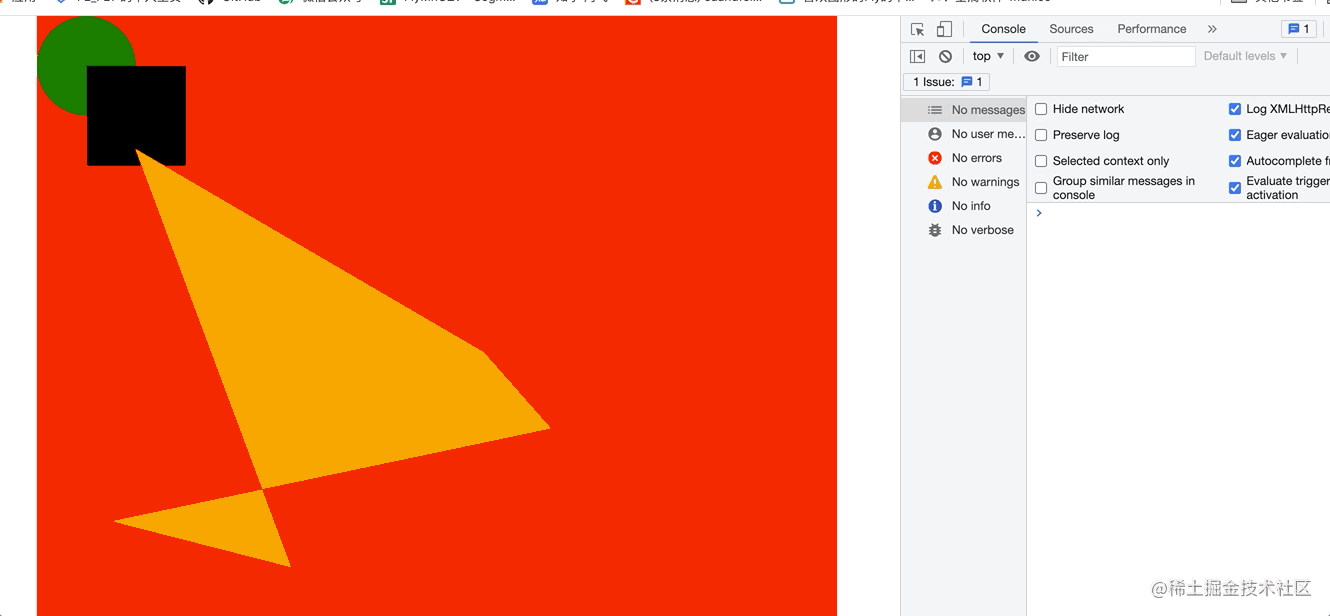``````circle.on(click, (event) => {
event.isStopBubble = true
console.log(event, 'circle')
})
rect.on(click, (event) => {
console.log(event, 'rect')
})